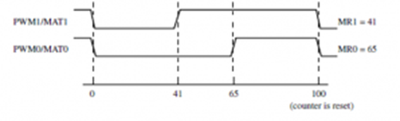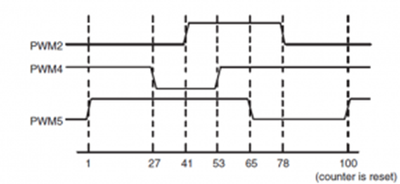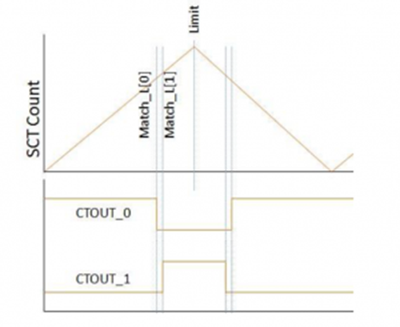# [LPC ALL] Analysis of the maximum number of PWM generated by SCTimer

cancel
Showing results for
Did you mean:

# [LPC ALL] Analysis of the maximum number of PWM generated by SCTimer

No ratings

## [LPC ALL] Analysis of the maximum number of PWM generated by SCTimer

Recently, customers reported that the number of PWM generated by SCTimer module was inconsistent between LPC55s06 user manual and data sheet. There are many kinds of PWM generation formats, so the maximum number of PWM generated by SCTimer is also different. I think the user manual and data sheet are not very clear, so this paper makes a specific analysis. It mainly depends on SCTimer resources, such as the number of events and output channels.

For all LPC series, the mechanism of SCTimer generating PWM is the same. Therefore, this paper takes LPC55s6 as an example.

LPC55s06 user manual:

• The SCTimer/PWM supports:
Eight inputs.
Ten outputs.
Sixteen match/capture registers.
Sixteen events.
Thirty two states.

According to the different control modes of generating PWM wave, this paper is divided into single-edge PWM control, dual-edge PWM control and center-aligned PWM control.

1. Single-edge PWM control

The figure below shows two single-edge control PWM waves with different duty cycles and the same PWM cycle length.It can be seen from the above figure that the two PWM waves require three events: when the counter reaches 41, 65 and 100 respectively. Because of the same PWM cycle length, all PWM outputs need only one period event.

Summary: The cycle length of all PWM waves are the same, so only one period event is required. The duty cycles of each PWM are different, and each PWM requires an event. The SCTimer of LPC55s06 has 16 events, one is used as PWM period event, and there are 15 left. Theoretically, 15 channels of PWM can be generated. However, LPC55s06 has only 10 outputs, so it can generate up to 10 single-edge control PWM waves.

2. Dual-edge PWM control

The figure below shows three Dual-edge control PWM waves with different duty cycles and the same PWM cycle length.It can be seen from the above figure that the three PWM waves require seven events: when the counter reaches 1, 27, 41, 53, 65, 78, 100.

Summary: PWM cycle length control needs one event, and each PWM duty cycle needs two events to trigger. The SCTimer of LPC55s06 has 16 events, one as PWM frequency event, and the remaining 15, so it can generate up to 7 dual-edge control PWM waves.

3. Center-aligned PWM control

Center-aligned PWM control is a special case of dual-edge PWM control. The figure below shows two center-aligned PWM waves with different duty cycles and the same PWM duty length.It can be seen from the above figure that the two center-aligned PWM waves need three events in total, which are the PWM cycle length and the duty cycle trigger of the two PWM waves. Because the left and right are symmetrical, only one event is needed to control the duty cycle of one PWM.

Summary: All PWM have the same cycle length, so an event is required. The duty cycle of each PWM circuit is different, but the left and right are symmetrical, and an event trigger is required for each circuit. The SCTimer of LPC55s06 has 16 events, one is used as PWM cycle length, and there are 15 left. Theoretically, 15 channels of PWM can be generated, but LPC55s06 has only 10 outputs, so it can generate up to 10 channels of unilateral control PWM wave.

Summary:

Maximum number of PWM generated by LPC55s6 SCTimer:

Single-edge PWM control: 10

Dual-edge PWM control: 7

Center-aligned control: 10

The number of SCTimer events and output channels is different with different chips, but the analysis method is the same. Customers can analyze whether the SCTimer in a certain chip meets the requirements.

Labels (5)

• ### LPC800

Version history
Revision #:
3 of 3
Last update:
‎03-22-2022 08:14 PM
Updated by: